# Optical Receiver Noise

Optical receivers convert incident optical power Pin into electric current through a photodiode. The relation Ip = RPin assumes that such a conversion is noise free. However, this is not the case even for a perfect receiver. Two fundamental noise mechanisms, shot noise and thermal noise, lead to fluctuations in the current even when the incident optical signal has a constant power. The relation Ip = RPin still holds if we interpret Ip as the average current. However, electrical noise induced by current fluctuations affects the receiver performance. The objective of this tutorial is to review the noise mechanisms and then discuss the signal-to-noise ratio (SNR) in optical receivers. The p-i-n and APD receivers are considered in separate subsections, as the SNR is also affected by the avalanche gain mechanism in APDs.

#### 1. Noise Mechanisms

The shot noise and thermal noise are the two fundamental noise mechanisms responsible for current fluctuations in all optical receivers even when the incident optical power Pin is constant. Of course, additional noise is generated if Pin is itself fluctuating because of noise produced by optical amplifiers. This tutorial considers only the noise generated at the receiver; optical noise is discussed in another tutorial.

Shot Noise

Shot noise is a manifestation of the fact that an electric current consists of a stream of electrons that are generated at random times. It was first studied by Schottky in 1918 and has been thoroughly investigated since then. The photodiode current generated in response to a constant optical signal can be written as

I(t) = Ip + is(t)

where Ip = RdPin is the average current and is(t) is a current fluctuation related to shot noise.

Mathematically, is(t) is a stationary random process with Poisson statistics (approximated often by Gaussian statistics). The autocorrelation function of is(t) is related to the spectral density Ss(f) by the Wiener-Khinchin theoremwhere angle brackets denote an ensemble average over fluctuations. The spectral density of shot noise is constant and is given by Ss(f) = qIp (an example of white noise). Note that Ss(f) is the two-sided spectral density, as negative frequencies are included in the equation. If only positive frequencies are considered by changing the lower limit of integration to zero, the one-sided spectral density becomes 2qIp.

The noise variance is obtained by setting τ = 0 in the equation above, i.e.,where Δf is the effective noise bandwidth of the receiver. The actual value of Δf depends on receiver design. It corresponds to the intrinsic photodetector bandwidth if fluctuations in the photocurrent are measured. In practice, a decision circuit may use voltage or some other quantity (e.g., signal integrated over the bit slot). One then has to consider the transfer functions of other receiver components such as the preamplifier and the low-pass filter. It is common to consider current fluctuations and include the total transfer function HT(f) by modifying the equation above as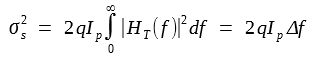where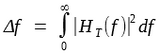and HT(f) is given by equation defined in the tutorial about optical receiver design. Since the dark current Id also generates shot noise, its contribution is included in the equation above by replacing Ip by Ip  + Id. The total shot noise is then given by

σs2 = 2q(Ip + Id)Δf

The quantity σs is the root-mean-square (RMS) value of the noise current induced by shot noise.

Thermal Noise

At a finite temperature, electrons move randomly in any conductor. Random thermal motion of electrons in a resistor manifests as a fluctuating current even in the absence of an applied voltage. The load resistor in the front end of an optical receiver adds such fluctuations to the current generated by the photodiode. This additional noise component is referred to as thermal noise. it is also called Johnson noise or Nyquist noise after the two scientists who first studied it experimentally and theoretically. Thermal noise can be included by modifying the first equation above as

I(t) = Ip + is(t) + iT(t)

where iT(t) is a current fluctuation induced by thermal noise. Mathematically, iT(t) is modeled as a stationary Gaussian random process with a spectral density that is frequency independent up to f ~ 1 THz (nearly white noise) and is given by

ST(f) = 2kBT/RL

where kB is the Boltzmann constant, T is the absolute temperature, and RL is the load resistor. As mentioned before, ST(f) is the two-sided spectral density.

The autocorrelation function iT(t) is given by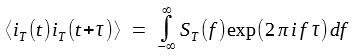The noise variance is obtained by setting τ = 0 and becomes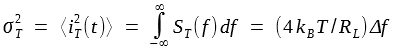where Δf is the effective noise bandwidth. The same bandwidth appears in the case of both shot and thermal noises. Note that σT2 does not depend on the average current Ip whereas σs2 does.

The equation above includes thermal noise generated in the load resistor. An actual receiver contains many other electrical components, some of which add additional noise. For example, noise is invariably added by electrical amplifiers. The amount of noise added depends on the front-end design and the type of amplifiers used.  In particular, the thermal noise is different for field-effect and bipolar transistors. considerable work has been done to estimate the amplifier noise for different front-end designs. A simple approach accounts for the amplifier noise by introducing a quantity Fn, referred to as the amplifier noise figure, and modifying the equation above as

σT2  = (4kBT/RL)FnΔf

Physically,  Fn represents the factor by which thermal noise is enhanced by various resistors used in pre- and main amplifiers.

The total current noise can be obtained by adding the contributions of shot noise and thermal noise. Since is(t) and iT(t) are independent random processes with approximately Gaussian statistics, the total variance of current fluctuations, ΔI = I - Ip = is + iT , can be obtained simply by adding individual variances. The result is

σ2 = <(ΔI)2> = σs2 + σT2 = 2q(Ip + Id)Δf + (4kBT/RL)FnΔf

This equation can be used to calculate the SNR of the photocurrent.

#### 2. p-i-n Receivers

The performance of an optical receiver depends on the SNR. the SNR of a receiver with a p-i-n photodiode is considered here; APD receivers are discussed in the following subsection. The SNR of any electrical signal is defined aswhere we used the fact that electrical power varies as the square of the current. By using the σ2 equation above together with Ip = RdPin , the SNR is related to the incident optical power aswhere Rd =  ηq/hν is the responsivity of the p-i-n photodiode.

Thermal-Noise Limit

In most cases of practical interest, thermal noise dominates receiver performance (σT2 >> σs2). Neglecting the shot-noise term in the equation above, the SNR becomesThus, the SNR varies as Pin2 in the thermal-noise limit. It can also be improved by increasing the load resistance. This is the reason why most receivers use a high-impedance or transimpedance front end. The effect of thermal noise is often quantified through a quantity called the noise-equivalent power (NEP). The NEP is defined as the minimum optical power per unit bandwidth required to produce SNR = 1 and is given by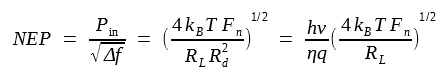Another quantity, called detectivity and defined as (NEP)-1, is also used for this purpose. The advantage of specifying NEP or the detectivity for a p-i-n receiver is that it can be used to estimate the optical power needed to obtain a specific value of SNR if the bandwidth Δf is know. Typical values of NEP are in the range 1-10 pw/Hz1/2.

Shot-Noise Limit

Consider the opposite limit in which the receiver performance is dominated by shot noise (σs2 >> σT2). Since σs2 increases linearly with Pin , the shot-noise limit can be achieved by making the incident power large. The dark current Id can be neglected in that situation. We then get the following expression for SNR:The SNR increases linearly with Pin in the shot-noise limit and depends only on the quantum efficiency η, the bandwidth Δf, and the photon energy hν. It can be written in terms of the number photons Np contained in the "1" bit. If we usefor the pulse energy of a bit of duration 1/B, where B is the bit rate, and note that Ep = Nphv, we can write Pin as Pin = NphvB. By choosing Δf = B/2 (a typical value for the bandwidth), the SNR per bit is simply given by ηNp. In the shot-noise limit, a SNR of 20 dB can be realized if Np = 100 and η ≈ 1. By contrast, several thousand photons are required to obtain SNR = 20 dB when thermal noise dominates the receiver. As a reference, for a 1.55-μm receiver operating at 10 Gb/s, Np = 100 when Pin ≈ 130 nW.

#### 3. APD Receivers

Optical receivers that employ an APD generally provide a higher SNR for the same incident optical power. The improvement is due to the internal gain that increases the photocurrent by a multiplication factor M so that

Ip = MRdPin = RAPDPin

where RAPD ≡ MRd is the APD responsivity, enhanced by a factor of M compared with that of p-i-n photodiodes. The SNR should improve by a factor M2 if the receiver noise were unaffected by the internal gain mechanism of APDs. Unfortunately, this is not the case, and the SNR improvement is considerably reduced.

Shot-Noise Enhancement

Thermal noise remains the same for APD receivers, as it originates in the electrical components that are not part of the APD. This is not the case for shot noise. The APD gain results from generation of secondary electron-hole pairs through the process of impact ionization. Since such pairs are generated at random times, an additional contribution is added to the shot noise associated with the generation of primary electron-hole pairs. In effect, the multiplication factor itself is a random variable, and M appearing in the equation above represents the average APD gain. Total shot noise is given bywhere FA is the excess noise factor of the APD and is given by

FA(M) = kAM + (1 - kA)(2 - 1/M)

The dimensionless parameter kA = αhe if αh < αe but is defined as kA = αeh when αh > αe. In other words, kA is in the range 0 < kA < 1. In general, FA increases with M. However, although FA is at most 2 for kA = 0, it keeps on increasing linearly (FA = M) when kA = 1. The ratio kA should be as small as possible for achieving the best performance from an APD.

If the avalanche-gain process were noise free (FA = 1), both Ip and σs would increase by the same factor M, and the SNR would be unaffected as far as the shot-noise contribution is concerned. In practice, the SNR of APD receivers is worse than that of p-i-n receivers when shot noise dominates because of the excess noise generated inside the APD. It is the dominance of thermal noise in practical receivers that makes APDs attractive. In fact, the SNR of APD receivers can be written as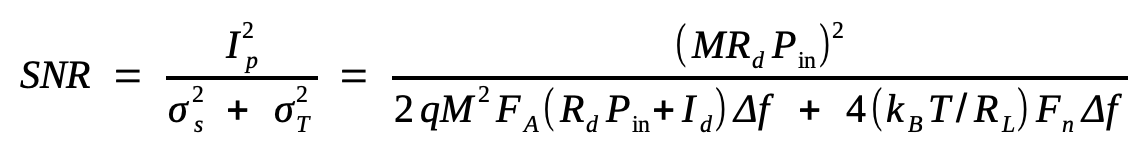The following figure shows the dependence of SNR on received power Pin for three values of APD gain M using Rd = 1 A/W, Id = 1 nA, kA = 0.7, and σT = 1 µA for a receiver with a 30-GHz bandwidth.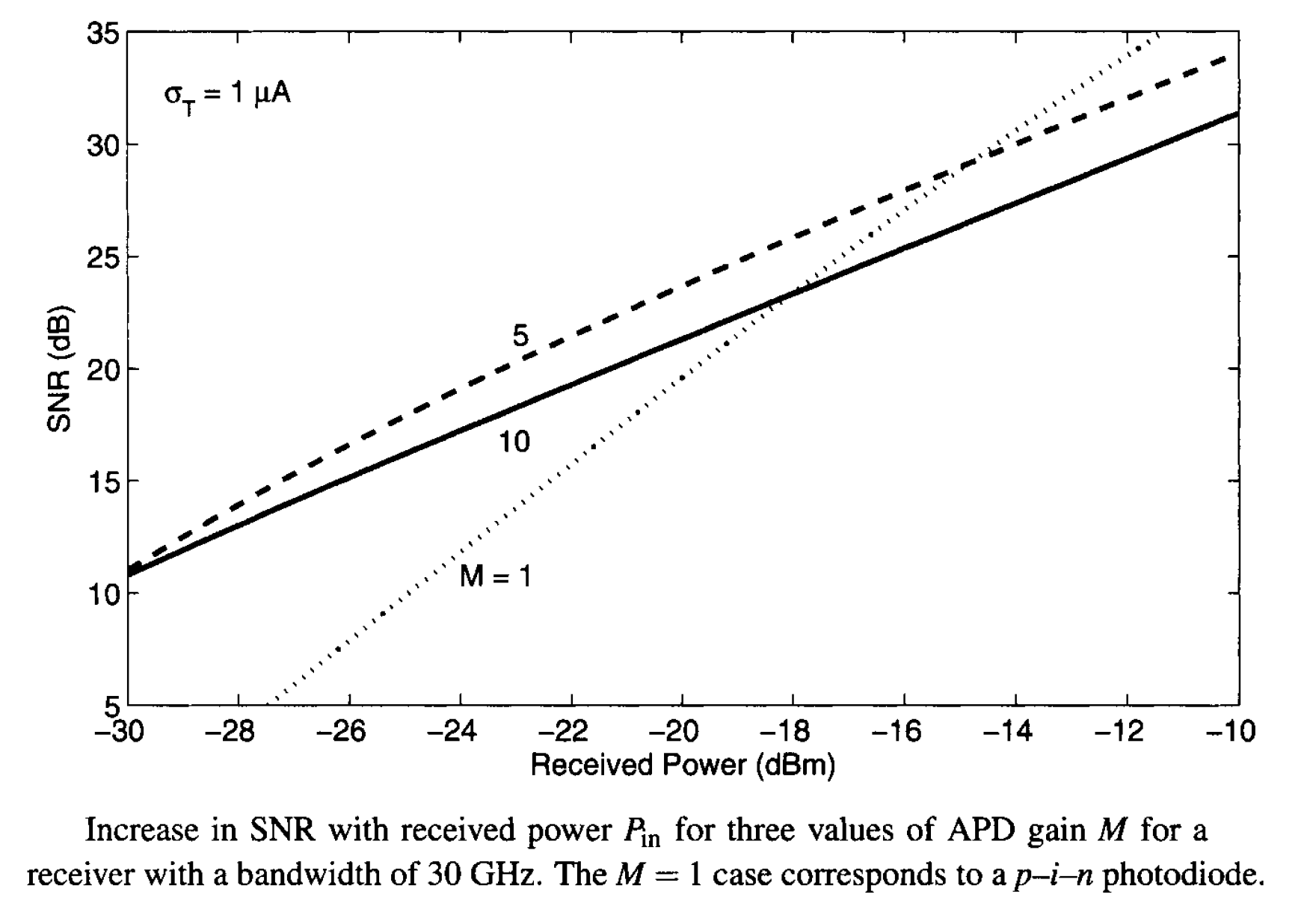Several features are noteworthy in this figure. Noting that M = 1 case corresponds to the use of a p-i-n photodiode, it is evident that the SNR is in fact degraded for an APD receiver when input powers are relatively large. Any improvement in SNR occurs only for low input power levels (below -20 dBm). The reason behind this behavior is related to the enhancement of shot noise in APD receivers. At low power levels, thermal noise dominates over shot noise, and the APD gain helps. However, as the APD gain increases, shot noise begins to dominate over thermal noise, and APD performs worse than a p-i-n photodiode under the same operating conditions. To make this point clear, we consider the two limits separately.

In the thermal-noise limit s << σT), the SNR becomes

SNR = (RLRd2/4kBTFnΔf)M2Pin2

and is improved, as expected, by a factor of M2 compared with that of p-i-n receivers. By contrast, in the shot-noise limit (σs >> σT), the SNR is given byand is reduced by the excess noise factor FA compared with that of p-i-n receivers.

Optimum APD Gain

The SNR equation shows that for a given Pin, the SNR of APD receivers is maximum for an optimum value Mopt of the APD gain M. It is easy to show that the SNR is maximum when Mopt satisfies the following cubic polynomial:The optimum value Mopt depends on a large number of the receiver parameters, such as the dark current, the responsivity R, and the ionization-coefficient ratio kA. However, it is independent of receiver bandwidth. The most notable feature of this equation is that Mopt decreases with an increase in Pin. The following figure shows the variation of Mopt with Pin for several values of kA by using typical parameter values RL = 1 kΩ, Fn = 2, R = 1 A/W, and Id = 2 nA corresponding to a 1.55-µm InGaAs receiver.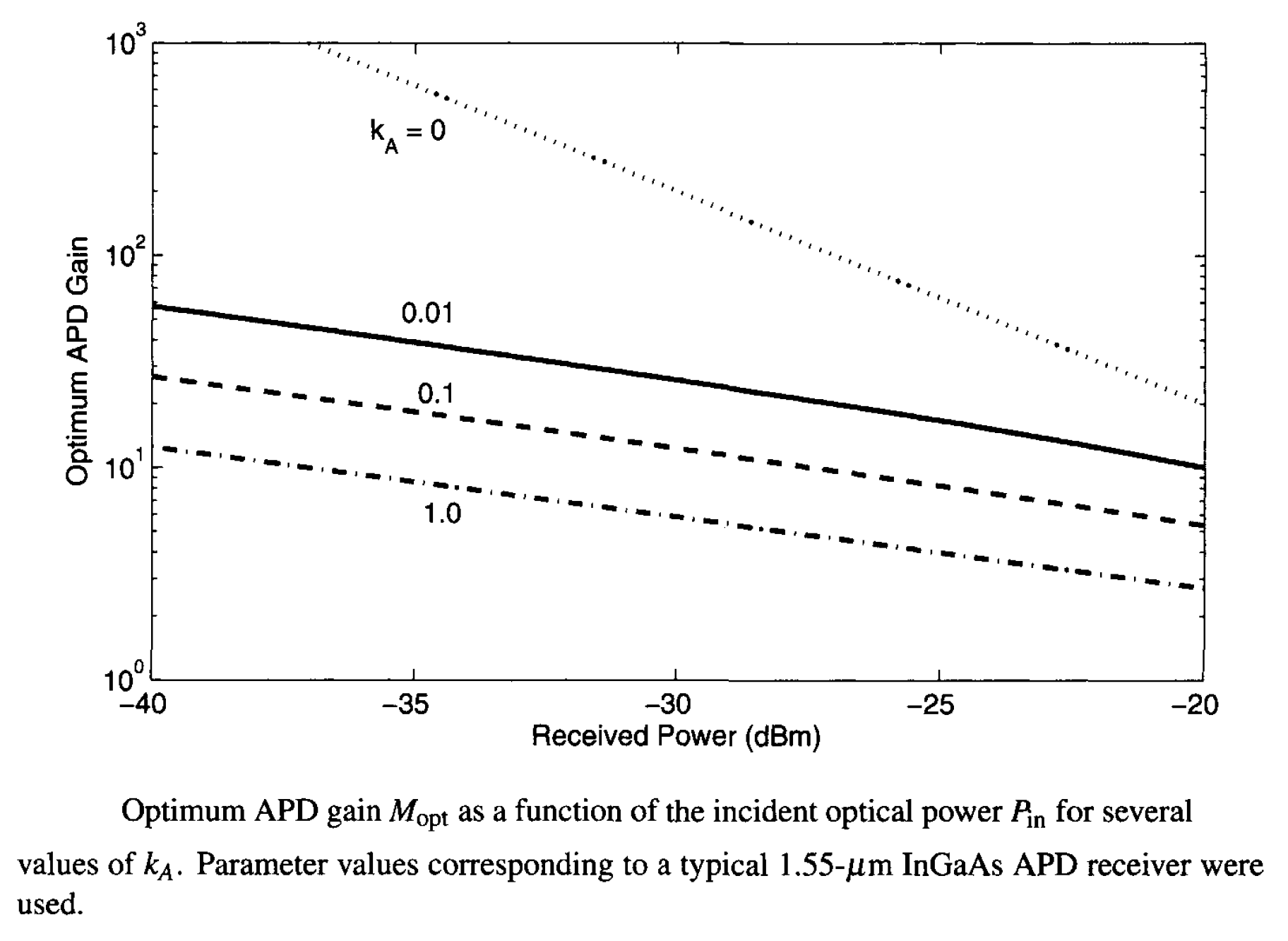The optimum APD gain is quite sensitive to the ionization-coefficient ratio kA. For kA = 0, Mopt decreases inversely with Pin, as can readily be inferred from the equation above by noting that the contribution of Id is negligible in practice. By contrast, Mopt varies as Pin-1/3 for kA = 1, and this form of dependence appears to hold even for kA as small as 0.01 as long as Mopt > 10. In fact, by neglecting the second term, Mopt is well approximated byfor kA in the range 0.01-1. This expression shows the critical role played by the ionization-coefficient ratio kA. For Si APDs, for which kA << 1, Mopt can be as large as 100. By contrast, Mopt is in the neighborhood of 10 for InGaAs receivers, since kA ≈ 0.7 for them. APD-based InGaAs receivers are useful for optical communication systems because of their higher sensitivity that allows them to operate at lower input power levels. However, with the advent of optical amplifiers, APDs are rarely employed in modern lightwave systems.